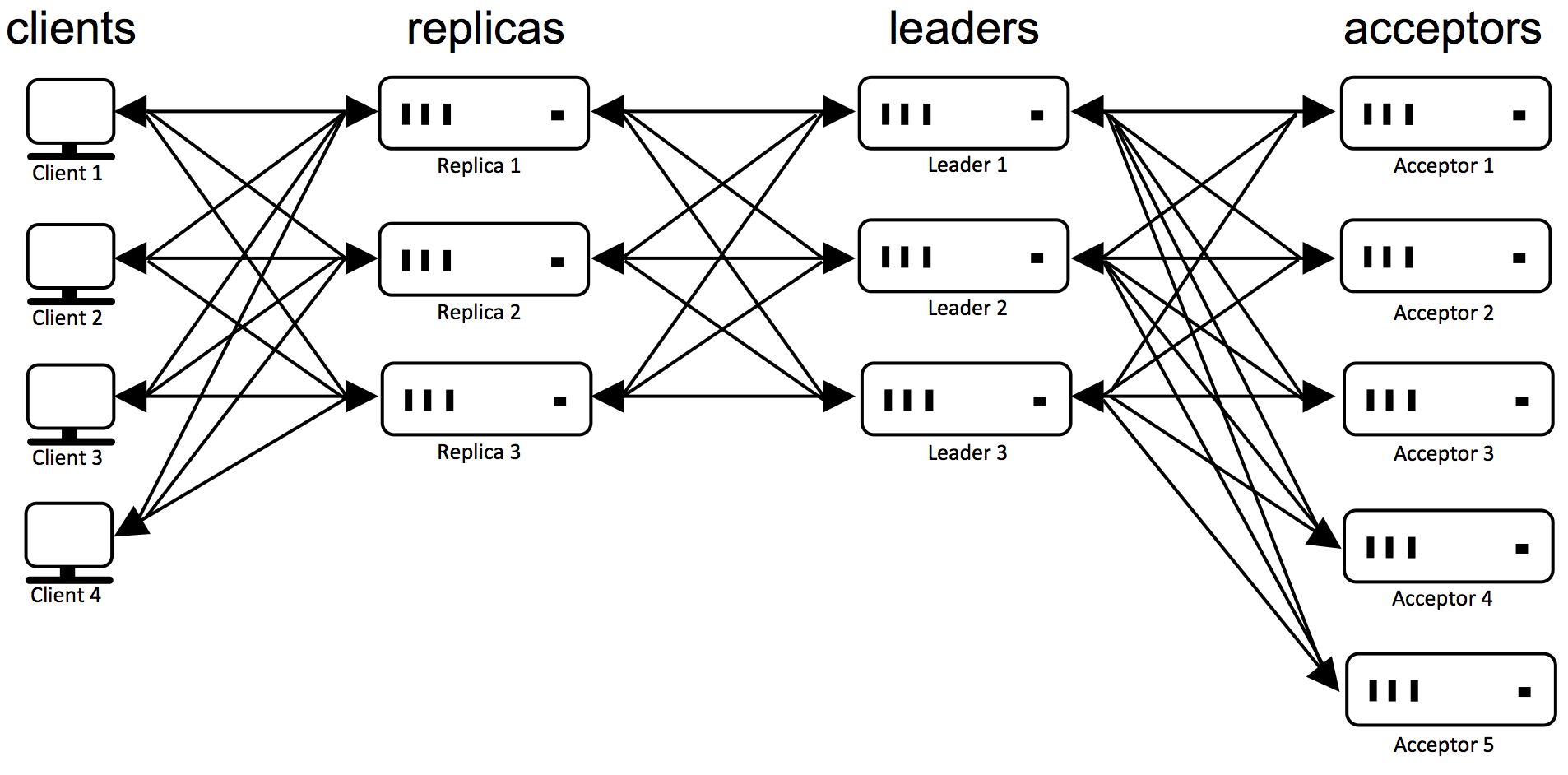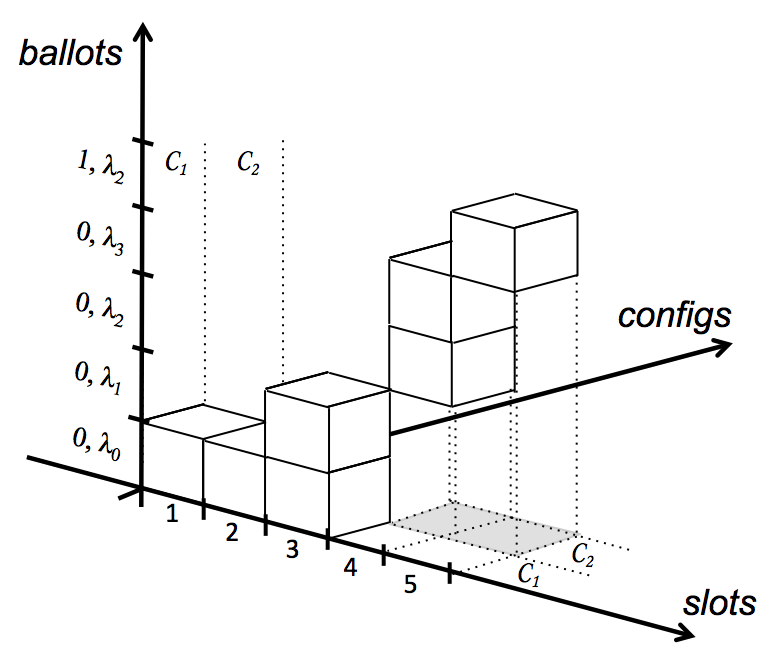# The Synod Protocol and Acceptors

In the Synod protocol, there is an unbounded collection of $ballots$. Ballots are the key to liveness properties in Paxos. Each ballot has a unique $leader$. A leader can be working on arbitrarily many ballots, although it will be predominantly working on one at a time. A leader process has a unique identifier called the $leader$ $identifier$. Each ballot has a unique identifier, called its $ballot$ $number$. Ballot numbers are totally ordered, that is, for any two different ballot numbers, one is before or after the other. Do not confuse ballot numbers and slot numbers; they are orthogonal concepts. One ballot can be used to decide multiple slots, and one slot may be targeted by multiple ballots.

In this description, we will have ballot numbers be lexicographically ordered pairs of an integer and its leader identifier (consequently, leader identifiers need to be totally ordered as well). This way, given a ballot number, it is trivial to see who the leader of the ballot is. We will use one special ballot number ⊥ that is ordered before any normal ballot number, but does not correspond to any ballot.

During the Synod protocol leaders send messages to acceptors, so one can think of acceptors as servers, and leaders as their clients. Acceptors maintain the fault tolerant memory of Paxos, preventing conflicting decisions from being made. Acceptors use a voting protocol, allowing a unanimous majority of acceptors to decide without needing input from the remaining acceptors. Thus, in order to tolerate $f$ crash failures, Paxos needs at least $2f + 1$ acceptors, always leaving at least $f + 1$ acceptors to maintain the fault tolerant memory. Keep in mind that acceptors are not replicas of one another, because they get different sequences of input from leaders.

The figure below illustrates the communication patterns between the various types of processes in a setting where $f = 2$.###### Acceptor State

An acceptor is quite simple, as it is passive and only sends messages in response to requests. Its state consists of two variables. Let a $pvalue$ be a triple consisting of a ballot number, a slot number, and a command. If $\alpha$ is the identifier of an acceptor, then the acceptor's state is described by

• $\alpha.ballot\_num$: a ballot number, initially $\bot$.
• $\alpha.accepted$: a set of pvalues, initially empty.

Under the direction of messages sent by leaders, the state of an acceptor can change. Let $p = \langle b, s, c \rangle$ be a pvalue consisting of a ballot number $b$, a slot number $s$, and a command $c$. When an acceptor $\alpha$ adds $p$ to $\alpha.accepted$, we say that $\alpha$ $accepts$ $p$. An acceptor may accept the same pvalue multiple times. When $\alpha$ sets its ballot number to $b$ for the first time, we say that $\alpha$ $adopts$ $b$.

###### Acceptor Invariants

Knowing these invariants is an invaluable help to understanding the Synod protocol:

• A1: An acceptor can only adopt strictly increasing ballot numbers.
• A2: An acceptor $\alpha$ can only accept $\langle b, s, c \rangle$ if $b = \alpha.ballot\_num$;
• A3: Acceptor $\alpha$ cannot remove pvalues from $\alpha.accepted$ (we will modify this impractical restriction later).
• A4: Suppose $\alpha$ and $\alpha'$ are acceptors, with $\langle b, s, c \rangle \in \alpha.accepted$ and $\langle b, s, c' \rangle \in \alpha'.accepted$. Then $c = c'$. Informally, given a particular ballot number and slot number, there can be at most one proposed command under consideration by the set of acceptors.
• A5: Suppose that for each $\alpha$ among a majority of acceptors, $\langle b, s, c \rangle \in \alpha.accepted$. If $b' > b$ and $\langle b', s, c' \rangle \in \alpha'.accepted$, then $c = c'$.

It is important to realize that Invariant A5 works in two ways. In one way, if all acceptors in a majority have accepted a particular pvalue $\langle b, s, c \rangle$, then any pvalue for a later ballot will contain the same command $c$ for slot $s$. In the other way, suppose some acceptor accepts $\langle b', s, c' \rangle$. At a later time, if any majority of acceptors accepts pvalue $\langle b, s, c \rangle$ on an earlier ballot $b < b'$, then $c = c'$.

###### Acceptor Operational Description

Below you can find the pseudo-code for an Acceptor:

$\texttt{process} ~ \textit{Acceptor}()$
$\texttt{var} ~ ballot\_num := \bot, accepted := \emptyset$;

$\texttt{for ever}$
$\texttt{switch} ~ \textit{receive}()$
$\texttt{case} ~ \langle \textbf{p1a}, \lambda, b \rangle:$
$\texttt{if} ~ b > ballot\_num ~ \texttt{then}$
$ballot\_num := b$;
$\texttt{end if}$
$\textit{send}(\lambda, \langle \textbf{p1b}, self(), ballot\_num, accepted \rangle)$;
$\texttt{end case}$
$\texttt{case} ~ \langle \textbf{p2a}, \lambda, \langle b, s, c \rangle \rangle:$
$\texttt{if} ~ b = ballot\_num ~ \texttt{then}$
$accepted := accepted \cup \{ \langle b, s, c \rangle \}$;
$\texttt{end if}$
$\textit{send}(\lambda, \langle \textbf{p2b}, self(), ballot\_num \rangle)$;
$\texttt{end case}$
$\texttt{end switch}$
$\texttt{end for}$
$\texttt{end process}$

The Acceptor runs in an infinite loop, receiving two kinds of request messages from leaders (note the use of pattern matching):

• $\langle \textbf{p1a}, \lambda, b \rangle$: Upon receiving a phase 1a'' request message from a leader with identifier $\lambda$, for a ballot number $b$, an acceptor makes the following transition. First, the acceptor adopts $b$ if and only if it exceeds its current ballot number. Then it returns to $\lambda$ a phase 1b'' response message containing its current ballot number and all pvalues accepted thus far by the acceptor.
• $\langle \textbf{p2a}, \lambda, \langle b, s, c \rangle \rangle$: Upon receiving a phase 2a'' request message from leader $\lambda$ with pvalue $\langle b, s, c \rangle$, an acceptor makes the following transition. If the current ballot number equals~$b$, then the acceptor accepts $\langle b, s, c \rangle$. The acceptor returns to $\lambda$ a phase 2b'' response message containing its current ballot number.
###### Maintenance of Acceptor Invariants

It is easy to see that the code enforces Invariants A1, A2, and A3. For checking the remaining two invariants, which involve multiple acceptors, we have to study what a leader does first, which is described in the following subsections.

An instance of the Synod protocol uses a fixed configuration $\mathcal{C}$ consisting of at least $f+1$ leaders and $2f+1$ acceptors. For simplicity, assume configurations have no processes in common. Instances follow each other, creating a reconfigurable protocol. The 3D graph below shows the relation between ballots, slots, and configurations. A leader can use a single ballot to decide multiple slots, as in the case for slots $1$ and $2$. Multiple leaders might use multiple ballots during a single slot, as shown in slot $3$. A configuration can have multiple ballots and can span multiple slots, but each slot and each ballot has only one configuration associated with it.In the Synod protocol slot numbers and ballot numbers are
orthogonal concepts. One ballot can be used to decide on multiple
slots, like in slot 1 and slot 2. A slot may be considered by
multiple ballots, such as in slot 3. A configuration can span
multiple ballots and multiple slots, but they each belong to a
single configuration.

According to Invariant A4, there can be at most one proposed command per ballot number and slot number. The leader of a ballot is responsible for selecting a command for each slot, in such a way that selected commands cannot conflict with decisions on other ballots (Invariant A5).